# JP2007301003A - Motor function testing apparatus and method for comparing phases between motor waveforms - Google Patents

Motor function testing apparatus and method for comparing phases between motor waveforms Download PDF

## Info

Publication number
JP2007301003A
JP2007301003A JP2006130124A JP2006130124A JP2007301003A JP 2007301003 A JP2007301003 A JP 2007301003A JP 2006130124 A JP2006130124 A JP 2006130124A JP 2006130124 A JP2006130124 A JP 2006130124A JP 2007301003 A JP2007301003 A JP 2007301003A
Authority
JP
Japan
Prior art keywords
motion
waveform
peak
plurality
waveforms
Prior art date
Legal status (The legal status is an assumption and is not a legal conclusion. Google has not performed a legal analysis and makes no representation as to the accuracy of the status listed.)
Abandoned
Application number
JP2006130124A
Other languages
Japanese (ja)
Other versions
JP2007301003A5 (en
Inventor
Akihiko Kandori
Takeshi Miyashita
Kuniomi Ogata

Original Assignee
Hitachi Ltd

Priority date (The priority date is an assumption and is not a legal conclusion. Google has not performed a legal analysis and makes no representation as to the accuracy of the date listed.)
Filing date
Publication date
Application filed by Hitachi Ltd, 株式会社日立製作所 filed Critical Hitachi Ltd
Priority to JP2006130124A priority Critical patent/JP2007301003A/en
Publication of JP2007301003A5 publication Critical patent/JP2007301003A5/ja
Publication of JP2007301003A publication Critical patent/JP2007301003A/en
Application status is Abandoned legal-status Critical

• 238000004458 analytical methods Methods 0 abstract 3

## Images

•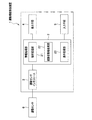•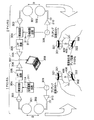•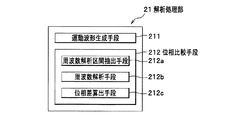•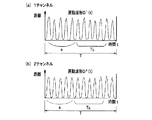•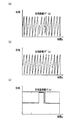•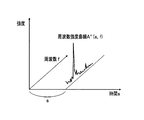•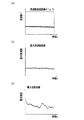••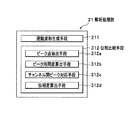•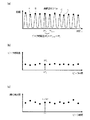•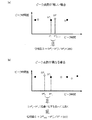•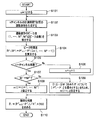•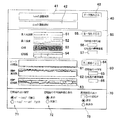•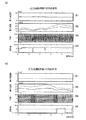•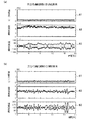•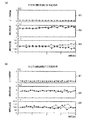## Classifications

• AHUMAN NECESSITIES
• A61MEDICAL OR VETERINARY SCIENCE; HYGIENE
• A61BDIAGNOSIS; SURGERY; IDENTIFICATION
• A61B5/00Detecting, measuring or recording for diagnostic purposes; Identification of persons
• A61B5/103Detecting, measuring or recording devices for testing the shape, pattern, colour, size or movement of the body or parts thereof, for diagnostic purposes
• A61B5/11Measuring movement of the entire body or parts thereof, e.g. head or hand tremor, mobility of a limb
• A61B5/1101Detecting tremor
• AHUMAN NECESSITIES
• A61MEDICAL OR VETERINARY SCIENCE; HYGIENE
• A61BDIAGNOSIS; SURGERY; IDENTIFICATION
• A61B5/00Detecting, measuring or recording for diagnostic purposes; Identification of persons
• A61B5/103Detecting, measuring or recording devices for testing the shape, pattern, colour, size or movement of the body or parts thereof, for diagnostic purposes
• A61B5/11Measuring movement of the entire body or parts thereof, e.g. head or hand tremor, mobility of a limb
• A61B5/1124Determining motor skills
• AHUMAN NECESSITIES
• A61MEDICAL OR VETERINARY SCIENCE; HYGIENE
• A61BDIAGNOSIS; SURGERY; IDENTIFICATION
• A61B5/00Detecting, measuring or recording for diagnostic purposes; Identification of persons
• A61B5/103Detecting, measuring or recording devices for testing the shape, pattern, colour, size or movement of the body or parts thereof, for diagnostic purposes
• A61B5/11Measuring movement of the entire body or parts thereof, e.g. head or hand tremor, mobility of a limb
• A61B5/1124Determining motor skills
• A61B5/1125Grasping motions of hands
• AHUMAN NECESSITIES
• A61MEDICAL OR VETERINARY SCIENCE; HYGIENE
• A61BDIAGNOSIS; SURGERY; IDENTIFICATION
• A61B5/00Detecting, measuring or recording for diagnostic purposes; Identification of persons
• A61B5/103Detecting, measuring or recording devices for testing the shape, pattern, colour, size or movement of the body or parts thereof, for diagnostic purposes
• A61B5/11Measuring movement of the entire body or parts thereof, e.g. head or hand tremor, mobility of a limb
• A61B5/1126Measuring movement of the entire body or parts thereof, e.g. head or hand tremor, mobility of a limb using a particular sensing technique
• AHUMAN NECESSITIES
• A61MEDICAL OR VETERINARY SCIENCE; HYGIENE
• A61BDIAGNOSIS; SURGERY; IDENTIFICATION
• A61B5/00Detecting, measuring or recording for diagnostic purposes; Identification of persons
• A61B5/68Arrangements of detecting, measuring or recording means, e.g. sensors, in relation to patient
• A61B5/6801Arrangements of detecting, measuring or recording means, e.g. sensors, in relation to patient specially adapted to be attached to or worn on the body surface
• A61B5/6813Specially adapted to be attached to a specific body part
• A61B5/6825Hand
• A61B5/6826Finger

## Abstract

A motor function test apparatus and a phase comparison method between motion waveforms capable of evaluating in detail the relationship between a plurality of motions.
A motor function testing apparatus comprising: analysis means for analyzing time-series waveform data acquired from a motion sensor; and display means for displaying an analysis result analyzed by the analysis means. The means 21 includes a phase comparison means 212 for comparing phases between a plurality of motion waveforms respectively acquired based on a plurality of waveform data.
[Selection] Figure 3

## Description

The present invention relates to a device for examining a motor function, and more particularly to a device for analyzing a motion waveform acquired by a motion sensor and displaying quantitative motion information, and a phase comparison method between motion waveforms.

A cerebral infarction refers to an infarct (ischemic brain parenchymal necrosis) in the perfusion region due to stenosis or occlusion of the cerebral feeding artery. In cerebral infarction, specific symptoms such as motor paralysis, sensory disturbance, ataxia, and abnormal eye movement appear corresponding to the lesion site. Conventionally, in order to diagnose cerebral infarction, diagnosis has been made with reference to examination findings such as X-ray CT, MRI, and cerebral angiography in addition to examination findings of such symptoms. However, with such a diagnostic method, it is difficult to quantitatively evaluate, for example, a decrease in motor function due to motor paralysis even if symptoms and infarct sites can be diagnosed.
Therefore, as a method for quantitatively evaluating motor function, for example, a method of tapping (hitting) a finger on a computer keyboard with respect to a subject has been studied (for example, see Non-Patent Document 1). According to the method described in Non-Patent Document 1, for a cerebral infarction patient with unilateral paralysis, finger tapping is performed with both hands, and an average value and standard deviation of the tapping interval are obtained, thereby quantitatively evaluating motor function. can do.
McCombe Waller S, Whitall J., "Fine Motor Control in Adults With and Without Chronic Hemiparesis: Baseline Comparison to Nondisabled Adults and Effects of Bilateral Arm Training" Arch Phys Med Rehabil 85, 1076-1083 (2004)

However, the method described in Non-Patent Document 1 has a problem in that it is impossible to know in detail the relevance of movement between both hands because it cannot analyze the tapping timing phase shift between both hands over time. It was.

Then, this invention makes it a subject to provide the motor function test | inspection apparatus which can evaluate the relationship of several exercise | movement in detail, and the phase comparison method between exercise | movement waveforms.

In order to achieve the above object, the present invention provides an exercise function comprising: analysis means for analyzing time-series waveform data acquired from a movement sensor; and display means for displaying an analysis result analyzed by the analysis means. An inspection apparatus, wherein the analysis means includes a phase comparison means for comparing phases between a plurality of motion waveforms respectively acquired based on the plurality of waveform data.

According to such a configuration, the phases between the plurality of motion waveforms can be compared, so that detailed evaluation of the relevance of the plurality of motions can be suitably performed.
Other inventions will be clarified in this specification.

According to the present invention, the relevance of a plurality of exercises can be evaluated in detail.

Hereinafter, the best mode for carrying out the present invention (hereinafter referred to as “embodiment”) will be described in detail with reference to the drawings as appropriate.

<< first embodiment >>
First, a first embodiment will be described with reference to the drawings. The first embodiment is an embodiment in which phases between a plurality of motion waveforms are compared by performing frequency analysis calculation on each of the plurality of motion waveforms.

FIG. 1 is a block diagram showing the overall configuration of the motor function testing device of the first embodiment. As shown in FIG. 1, the motor function testing device 1 includes an information processing unit 2, a motion sensor interface 3, a display unit 4, and an input unit 5.

The motion sensor 6 that acquires the motion information of the subject as waveform data is connected to the motor function testing device 1 via the motion sensor interface 3. The motion sensor 6 detects the motion information of the subject in time series, and can acquire the motion information of the subject corresponding to at least one of distance, speed, acceleration, and jerk as waveform data. If it is.

Here, the “subject” is an object to be measured by the motion sensor 6 and may be any object that operates, for example, a machine, an animal, a human, and the like. Unless otherwise specified in the present embodiment, it is assumed that the subject is a patient having a motor function disorder such as a cerebral infarction patient, Parkinson's disease patient, cervical spondylosis patient, etc. Yes.

FIG. 2 is a block diagram showing an example of the configuration of the motion sensor 6 in the present embodiment. As shown in FIG. 2, for example, the motion sensor 6 is a magnetic sensor type tapping device, and waveform data acquired by each of the tapping devices (1 channel, 2 channel) having the same configuration mounted on both hands of the subject is stored. Output to one computer 8. Therefore, in the present embodiment, a description will be given mainly of a one-channel tapping device as the motion sensor 6, and a duplicate description for the first and second channels is omitted.

In FIG. 2, the transmitting coil 302 is mounted on the back side of the thumb, and the receiving coil 301 is mounted on the back side of the index finger. The transmitting coil 302 is wound around the coil mounting member 322 and is connected to the current generating amplifier 310. The receiving coil 301 is wound around the coil mounting member 321 and is connected to the preamplifier circuit 303.
The coil attachment members 322 and 321 around which the transmission coil 302 and the reception coil 301 are wound are attached to respective fingers via bands 406 and 405 made of elastically deformable members such as rubber and sponge, for example. ing.

Then, an AC voltage having a specific frequency (for example, 20 kHz) is created by the AC generation circuit 309. The AC voltage having a specific frequency generated by the AC generation circuit 309 is converted into an AC current having a specific frequency by the current generation amplifier circuit 310, and the AC current generated by the current generation amplifier circuit 310 is used for transmission. It is configured to flow through the coil 302. The magnetic field generated by the transmission coil 302 generates an induced electromotive force in the reception coil 301.

The generated electromotive force (having the same frequency as the AC voltage having a specific frequency created by the AC generation circuit 309) is amplified by the preamplifier circuit 303, and the amplified signal is input to the detection circuit 304. The
Since the detection circuit 304 performs detection at a specific frequency or a double frequency generated by the AC generation circuit 309, the output of the AC generation circuit 309 is detected as a reference signal 311A after the phase is adjusted by the phase adjustment circuit 311. The reference signal input terminal of the circuit 304 is connected.

In addition, the phase adjustment circuit 311 is not necessarily required when detecting at a frequency twice the specific frequency. As a simple circuit configuration for detecting at a double frequency, a specific frequency of the AC generation circuit 309 is set to a double frequency, converted to a half frequency by a frequency divider, and then input to the current generation amplifier circuit 310. In the reference signal 311A, a signal having a frequency twice the specific frequency of the AC generation circuit 309 is connected to the reference signal input terminal of the detection circuit 304.

The output of the detection circuit 304 is passed through an LPF (Low-Pass filter) circuit 305, amplified by an amplifier circuit 306 to obtain a desired voltage, and then an output 307 is obtained. The output 307 is a voltage corresponding to the relative distance D between the receiving coil 301 and the transmitting coil 302 attached to the subject.
The output 307 is converted into digital data by an analog / digital conversion board (AD board) built in the computer 308 and input to the computer 308.

Here, in the present embodiment, for example, a task is given to the subject to perform a tapping motion for overlapping the ventral sides of the index finger and the thumb with both hands simultaneously as fast as possible for 20 seconds (synchronous motion). In addition, a task is given to the subject, for example, to perform a tapping motion that overlaps the ventral sides of the index finger and the thumb alternately with both hands as quickly as possible for 20 seconds (asynchronous motion).
And the motion sensor 6 of this embodiment shall acquire the motion information at this time as waveform data which can be converted into a distance waveform. That is, in this embodiment, in any task, two waveform data measured by the 1-channel and 2-channel tapping devices are acquired. Therefore, in the present embodiment, description will be made assuming that two waveform data measured in parallel in the same time zone by a tapping device consisting of one channel and two channels as the “plurality” of waveform data. The “plurality” of waveform data is not limited to this.

The information processing unit 2 shown in FIG. 1 analyzes the waveform data acquired by the motion sensor 6 to extract motion feature amounts, and appropriately displays the extracted feature amounts on the display unit 4 together with subject information and the like. It is what is displayed.

The motion sensor interface 3 includes, for example, an analog-digital conversion board (hereinafter referred to as “AD board”) provided in a general computer, and the waveform data of the analog signal detected by the motion sensor 6 is obtained at a predetermined sampling frequency S f . This is converted into waveform data of a digital signal and input to the information processing unit 2.
The sampling frequency S f is also used when extracting a frequency analysis section from the motion waveform, as will be described later.

The display unit 4 displays the subject information and exercise information processed by the information processing unit 2 and can be realized by, for example, an LCD (Liquid Crystal Display) or the like.

The input means 5 is used by an operator of the motor function testing apparatus 1 (not shown) for inputting subject information and instructing measurement and analysis, and can be realized by a keyboard, a mouse, or the like. it can. In addition, when inputting subject information or instructing measurement or analysis, an input screen may be displayed on the display unit 4 as a user interface for assisting input by the operator.

The information processing unit 2 includes an analysis processing unit 21, a subject information processing unit 22, and a display processing unit 23. The information processing unit 2 includes a CPU (Central Processing Unit), a memory including a ROM (Read Only Memory), a RAM (Random Access Memory), and a hard disk device. Each of the processing units 21 to 23 in the information processing unit 2 described above can be realized by the CPU reading a program or data stored in the memory or the hard disk device to the memory and executing arithmetic processing.

(Analysis processing section)
FIG. 3 is a block diagram illustrating a configuration of the analysis processing unit 21 according to the first embodiment. The analysis processing unit 21 extracts a motion feature amount based on the waveform data input from the motion sensor 6. The result analyzed by the analysis processing unit 21 is recorded in a subject data DB (not shown) provided in the subject information processing unit 22, and is appropriately read from the subject data DB by the display processing unit 23 and displayed on the display unit 4. .
Here, as shown in FIG. 3, the analysis processing unit 21 includes a motion waveform generation unit 211 and a phase comparison unit 212.

[Motion waveform generation means]
The waveform data acquired from the motion sensor 6 does not directly represent the motion waveform but is a voltage output that can be converted into the motion waveform.
The motion waveform generation means 211 converts the waveform data, which is the voltage output, into a corresponding motion waveform, and performs time differentiation or time integration on the converted motion waveform, thereby obtaining a distance waveform, a velocity waveform, and an acceleration waveform. The jerk waveform is generated in a complementary manner.

Here, the “movement waveform” can be converted into a distance waveform, a velocity waveform, an acceleration waveform, a jerk waveform, and the four types of movement waveforms (distance waveform, velocity waveform, acceleration waveform, jerk waveform), respectively. At least one of the waveform data is included. That is, the motion waveform to be analyzed by the motion function testing device 1 of the present embodiment can be acquired based on the waveform data measured by the motion sensor 6, and is measured by the motion sensor 6, for example. The waveform data itself may be used, or the four types of motion waveforms converted and complementarily generated from the waveform data may be used.
The time width T of these motion waveforms is usually the time measured by the motion sensor 6. For example, when the tapping motion of the subject is measured for 20 seconds, the time width T of the motion waveform is 20 seconds.

[Phase comparison means]
The phase comparison unit 212 compares phases between a plurality of motion waveforms respectively acquired based on a plurality of waveform data.
In the first embodiment, the phase comparison unit 212 performs a frequency analysis calculation on each of the plurality of motion waveforms to calculate the phase of the frequency at which the frequency intensity is maximum (hereinafter referred to as “maximum frequency”), By comparing the phases of the maximum frequencies, the phases of a plurality of motion waveforms are compared.
Here, the phase comparison unit 212 of the first embodiment includes a frequency analysis section extraction unit 212a, a frequency analysis unit 212b, and a phase difference calculation unit 212c.

<Frequency analysis section extraction means>
The frequency analysis section extraction unit 212a extracts a frequency analysis section having a predetermined time width T 0 to be subjected to frequency analysis by the frequency analysis unit 212b in the motion waveform.
At this time, the accuracy of frequency analysis in each frequency analysis section improves as the time width T 0 of the frequency analysis section to be extracted is increased. On the other hand, the shorter the time width T 0 of the frequency analysis section to be extracted, the more frequency analysis sections can be extracted per one motion waveform, so the information such as the phase of the maximum frequency that is the result of the frequency analysis can be obtained. It can be calculated finely over time. Therefore, it is preferable that an appropriate length is appropriately selected as the time width T 0 of the frequency analysis section to be extracted. In the present embodiment, for example, a frequency analysis section is assumed to be 10 seconds for a motion waveform of 20 seconds.

Here, with reference to FIG. 4, a procedure in which the frequency analysis section extraction unit 212a extracts a frequency analysis section from a distance waveform as an example of a motion waveform will be described. FIG. 4 is a conceptual diagram for explaining a procedure for extracting a plurality of frequency analysis sections from a distance waveform. FIG. 4 (a) is a distance waveform acquired by one channel, and FIG. 4 (b) is two channels. The distance waveform acquired by (1) is shown.
Note that distance waveform analysis can be applied to other motion waveform analysis in the same way, so in the following explanation, the word “motion waveform”, which is the superordinate concept, is used instead of “distance waveform”. I will explain. Further, in the 1-channel motion waveform D 1 (t) and the 2-channel motion waveform D 2 (t), the procedure for extracting the frequency analysis section is the same, so that D 1 (t) and D 2 (t) are particularly the same. The movement waveform D n (t) will be described without distinction between the two.

First, the frequency analysis section extraction unit 212a discretely displays the motion waveform D n (t). Here, the discretely displayed motion waveform D n i can be expressed by the following equation (1).

D n (t) = D n i (1)
(N = channel number)
(I = 1,..., L T )
(Time point of motion waveform L T
= Time width of motion waveform T × Sampling frequency S f )

In FIG. 4, the motion waveform D n (t) is shown, but when the frequency analysis section is actually extracted, the discretized motion waveform D n i is used.

Next, the frequency analysis section extraction unit 212a extracts frequency analysis sections D n u and i having a predetermined time width T 0 from the discretized motion waveform D n i . Here, the extracted frequency analysis sections D n u and i can be expressed by the following equation (2).
D n u , i = D n j (2)
(I = 1,..., L T0 )
(J = u + i)
(Time points u until frequency analysis interval
= Time width to frequency analysis interval s × S f )
( Number of time points in frequency analysis section L T0
= Time width of frequency analysis section T 0 × S f )

That is, in the expression (2), the frequency analysis sections D n u and i having the predetermined time width T 0 are extracted while being shifted by the time (minute time) of the 1 / sampling frequency S f .
The frequency analysis sections D n u and i extracted by the frequency analysis section extraction unit 212a are output to the frequency analysis unit 212b.

<Frequency analysis means>
Frequency analysis means 212b, each frequency analysis section D n u is extracted, performs frequency analysis of i, and calculates the phase of the maximum frequency in each frequency analysis section D n u, i.
Here, a procedure in which the frequency analysis unit 212b performs frequency analysis in the frequency analysis sections D n u and i will be described.
First, the frequency analysis unit 212b calculates the frequency intensities A n u and k and the phases Θ n u and k in the respective frequency analysis sections D n u and i by, for example, digital Fourier transform. Here, the digital Fourier transform process can be expressed by the following equation (3).

Frequency analysis section D n u , i → frequency intensity A n u , k , phase Θ n u , k (3)
(K = 1, Lf )
(Number of discrete points of frequency L f = L T0 / 2)
(K / T 0 = frequency)

Next, the frequency analysis unit 212b obtains the frequency intensities A n u and k and the phases Θ n u and k for all u satisfying [0 ≦ u ≦ L T −L T0 ] in the expression (3).
Then, the frequency analysis unit 212b searches for the frequency k that maximizes the frequency intensity A n u , k for each time u, and sets k (u).
Then, the frequency analysis means 212b is a phase Θ n u, k (u) at frequency k (u), the phase theta n u of the maximum frequency at each time u. In this maximum frequency phase Θ n u , u is a discretized time width (start time of the frequency analysis interval) s up to the frequency analysis interval as shown in the equation (2), so Θ n u = Θ n (s), which can be expressed as a phase curve Θ n (s) as shown in FIGS. FIG. 5A shows a phase curve Θ 1 (s) for one channel, and FIG. 5B shows a phase curve Θ 2 (s) for two channels.
Here, the phase Θ n (s) of the maximum frequency calculated by the frequency analysis unit 212b is output to the phase difference calculation unit 212c.

<Phase difference calculating means>
The phase difference calculation means 212c compares the phase Θ n (s) of the maximum frequency obtained for each of the plurality of motion waveforms D n (t), and calculates the phase difference Θ (s) of the maximum frequency between the plurality of motion waveforms. Is to be calculated.
Here, for example, when the phases between two motion waveforms acquired using the motion sensor 6 including the 1-channel and 2-channel tapping devices are compared as in the present embodiment, the phase difference Θ ( s) can be obtained by the following equation (4).

Maximum frequency phase difference Θ (s)
= Phase of maximum frequency of 2 channels Θ 2 (s)
−1 channel maximum frequency phase Θ 1 (s) (4)

This maximum frequency phase difference Θ (s) can be expressed as a phase difference curve Θ (s) as shown in FIG.
In addition, the phase difference Θ (s) of the maximum frequency between three or more motion waveforms is, for example, based on the phase of the maximum frequency of one motion waveform (for example, D 1 (t)) as another motion waveform ( For example, it can be obtained by calculating the difference between the phase of the maximum frequency of D 2 (t) and D 3 (t)).

Not only the above-described maximum frequency phase Θ n (s) but also the maximum frequency and the intensity of the maximum frequency can be calculated by frequency analysis such as general digital Fourier transform performed by the frequency analysis unit 212b. In other words, if the motor waveform is analyzed using the motor function test apparatus 1 of the first embodiment, the frequency intensity curve An (s, f) for each minute time as shown in FIG. 6 for each motor waveform. Can be obtained. The frequency analysis unit 212b can calculate not only the phase of the maximum frequency but also various feature quantities of motion using the frequency intensity curve An (s, f) for each minute time. For example, the frequency analysis unit 212b calculates the time corresponding to the frequency analysis section (for example, the time width s to the frequency analysis section), the frequency f, and the frequency intensity from the frequency intensity curve An (s, f) for each minute time. The feature amount can be read out, and further, the feature amount of the maximum frequency, the intensity of the maximum frequency, and the time feature amount corresponding to the frequency analysis section can be calculated from the frequency intensity curve An (s, f).

(Subject Information Processing Department)
Returning to FIG. 1, the subject information processing unit 22 includes a subject data DB (not shown) for recording information such as subject information and analysis results, and manages information recorded in the subject data DB.
More specifically, the subject information processing unit 22 1) registration, correction, deletion and search, sorting of subject information, 2) association of subject information with a motion waveform, 3) registration and correction of an analysis result of a motion waveform, Deletion (addition, correction, and deletion of items), 4) When statistical processing is performed, processing of the four items mainly for registration, correction, and deletion of the statistical processing results is performed in cooperation with the subject data DB.
The subject information registered in the subject data DB includes subject ID, name, date of birth, age, height, weight, disease name, comments on the subject, and the like.
The information management by the subject information processing unit 22 can be easily realized by a conventionally known program and data configuration.
The subject data DB can be realized by a hard disk device or the like.

(Display processing part)
The display processing unit 23 displays on the display means 4 information such as subject information registered in the subject data DB and analysis results of the motion waveform in a display format that is easy to visually understand using graph and table formats as appropriate. It is something to be made. The display processing unit 23 not only creates and displays the phase curve Θ n (s) and the phase difference curve Θ (s) shown in FIG. 5 but also displays the time corresponding to the frequency analysis section (for example, frequency analysis). A correlation diagram including at least two feature quantities of the feature quantity of frequency f and frequency intensity, or a time s corresponding to the maximum frequency, the intensity of the maximum frequency, and the frequency analysis section. A correlation diagram including at least two of the feature quantities can be created and displayed.

FIG. 7A is a graph showing a correlation between three feature amounts of a time width s, a frequency f, and a frequency intensity up to the frequency analysis section. In the graph shown in FIG. 7A, the intensity of the frequency is indicated by the intensity of the luminance in the actual display screen in which the intensity of the intensity is shown in color instead of black and white. FIG. 7B is a graph showing a correlation between two feature amounts of the time width s to the frequency analysis section and the maximum frequency. FIG. 7C is a graph showing the correlation between two feature amounts, the time width s to the frequency analysis section and the intensity of the maximum frequency (denoted as maximum intensity in the figure).

[Phase comparison method]
Next, referring to FIG. 8 with reference to FIG. 3, an example of a method for comparing phases of a plurality of motion waveforms using the motor function testing device 1 of the first embodiment will be described. FIG. 8 is a flowchart for explaining the phase comparison process of the motor function testing device 1 according to the first embodiment.

First, the motion waveform generation means 211 of the analysis processing unit 21 sets n = 1 when analyzing the waveform data acquired from the n-channel motion sensor 6 (see FIG. 1) (step S01).

Next, the motion waveform generation means 211 of the analysis processing unit 21 generates a motion waveform having a time width T based on the n-channel waveform data (step S02). As described above, the time width T is usually a measurement time by the motion sensor 6.

Then, the phase comparison unit 212 of the analysis processing unit 21 sets s = 0 seconds when the frequency analysis section extraction unit 212a extracts the frequency analysis section after s seconds from the start of the motion waveform (step S03).

Then, the phase comparison unit 212 of the analysis processing unit 21 uses the frequency analysis interval extraction unit 212a to extract a frequency analysis interval having a time width T 0 after s seconds from the start of the motion waveform (step S04).

Then, the phase comparison unit 212 of the analysis processing unit 21 performs frequency analysis calculation on the extracted frequency analysis section by the frequency analysis unit 212b, and calculates the phase Θ n (s) of the maximum frequency (step S05). For example, this frequency analysis calculation is a digital Fourier transform.

Then, the phase comparison unit 212 of the analysis processing unit 21 determines whether or not s ≦ T−T 0 by the frequency analysis unit 212b (step S06), and if s ≦ T−T 0 (Yes in step S06). ), S = s + 1 / S f (step S07), the process returns to step S04, and step S04 is performed until the phase Θ n (s) of the maximum frequency is calculated for all times s satisfying 0 ≦ s ≦ T−T 0. Repeat ~ S07. On the other hand, if s> T−T 0 (No in step S06), the process proceeds to step S08.

Then, the motion waveform generation means 211 of the analysis processing unit 21 determines whether n = total number of channels (step S08). If n = total number of channels is not satisfied (No in step S08), n = n + 1 is set (step S09). Returning to step S02, steps S02 to S09 are repeated until n = the total number of channels. On the other hand, if n = total number of channels (YES in step S08), the process proceeds to step S10.

Then, the phase comparison unit 212 of the analysis processing unit 21 calculates the phase difference Θ (s) of the maximum frequency between channels by the phase difference calculation unit 212c (step S10). As described above, for example, when the total number of channels is two, it can be calculated by the equation (4).

<< Second Embodiment >>
Next, a second embodiment will be described with reference to the drawings. In the second embodiment, peak points are extracted from a plurality of motion waveforms, and phases between the plurality of motion waveforms are compared based on a time difference between the peak points.
This second embodiment has a characteristic configuration in the phase comparison means in the analysis processing unit 21 as compared with the first embodiment. Therefore, in the description of the second embodiment, the phase comparison unit 312 of the analysis processing unit 21 will be described in detail, but the description overlapping with the first embodiment is omitted.

(Analysis processing section)
FIG. 9 is a block diagram illustrating a configuration of the analysis processing unit 21 according to the second embodiment. The analysis processing unit 21 of the second embodiment includes a motion waveform generation unit 211 and a phase comparison unit 312.
Further, the phase comparison means 312 of the second embodiment includes a peak point extraction means 312a, a peak time difference calculation means 312b, an inter-channel peak correspondence means (corresponding to a motion waveform peak correspondence means) 312c, and a phase difference calculation. Means 312d.

<Peak point extraction means>
The peak point extraction means 312a extracts peak points (1,..., M n ; M n is the number of peak points) in the motion waveform. In FIG. 10A, the peak point where the motion value (distance value) is a predetermined value or more is indicated by a black circle (●), but a peak point (not shown) having a predetermined value or less may be extracted. Moreover, it is good also as a structure which extracts both the peak point more than predetermined value and below predetermined value.
The peak points (1,..., M n ) extracted by the peak point extraction unit 312a are output to the peak time difference calculation unit 312b and the inter-channel peak correspondence unit 312c.

<Peak time difference calculation means>
The peak time difference calculation means 312b calculates a peak time difference composed of the time differences between temporally adjacent peak points in one motion waveform. In FIG. 10A, the temporally adjacent peak points are, for example, A and B, or C and D. The peak time difference R n i can be obtained by the following equation (5).

R n i = P n i + 1 −P n i (i = 1,..., M n −1) (5)

FIG. 10B is a graph plotting the correlation between the peak time difference R n i and the peak time (for example, P n i ) at one of the peak points used to calculate the peak time difference R n i .
FIG. 10C is a graph plotting the correlation between 1 / peak time difference R n i and the peak time (for example, P n i ) of one peak point used for calculating the peak time difference R n i . It is. Here, the 1 / peak time difference R n i corresponds to an instantaneous frequency at the peak time (hereinafter referred to as “instantaneous frequency”).
Then, the peak time difference R n i calculated by the peak time difference calculating unit 312b is output to the phase difference calculating unit 312d.

<Channel peak measure>
The inter-channel peak correspondence means 312c associates peak points among a plurality of motion waveforms.
Here, the procedure in which the peak correspondence unit 312c between channels associates the peak points between the two motion waveforms acquired in the first channel and the second channel will be described with reference to FIG. FIG. 11 is a diagram for explaining a procedure for associating a peak point between two motion waveforms, and shows a peak time difference-peak time plot (see FIG. 10B) generated in each of the two motion waveforms. FIG.
FIG. 11A shows a case where the number of peak points M n is the same in the two motion waveforms, and FIG. 11B shows a case where the number of peak points M n is different in the two motion waveforms. In FIG. 11, black circles (●) are peak points in the motion waveform of one channel, and white circles (◯) are peak points in the motion waveform of two channels.

As shown in FIG. 11 (a), when the number of peak points Mn extracted in the two motion waveforms is the same (three peak points in FIG. 11 (a)), the inter-channel peak correspondence means 312c. Associates peak points between motion waveforms in time order.
Specifically, in FIG. 11A, the inter-channel peak correspondence means 312c sets P 2 m (i by setting m (i) = i (i = 1,..., M 1 ). ) And P 1 i .
Here, the information on the peak point corresponding to the channel is output to the phase difference calculating means 312d.

Further, as shown in FIG. 11B, when the number of peak points M n extracted in the two motion waveforms are different (in FIG. 11B, four peak points (●) and three peak points (◯)). ), The inter-channel peak correspondence means 312c first sets the peak point of one motion waveform (for example, the peak point (●) of one channel) as the reference peak point, and the peak point ( For example, the peak point (◯) of channel 2 is set as the comparative peak point.
Next, the inter-channel peak correspondence means 312c calculates a time difference from each comparison peak point (O) for each reference peak point (●), and makes a comparison such that the time difference is the smallest with respect to the reference peak point (●). By selecting a peak point (◯) for each reference peak point (●), the peak points between the motion waveforms are made to correspond.
Specifically, the peak corresponding unit 312c is between the channels, in FIG. 11 (b), | P 2 j -P 1 i | a a a minimum j is set to m (i), and P 2 m (i) Correspond to P 1 i .
Here, the information on the peak point corresponding to the channel is output to the phase difference calculating means 312d.

In addition, although FIG. 11 demonstrated the case where the peak point between two motion waveforms was matched, the peak point between three or more motion waveforms can also be matched similarly.
For example, when the number of peak points M n extracted in three or more motion waveforms is the same, the inter-channel peak correspondence means 312c associates the peak points between the motion waveforms in time order as in FIG.

Further, for example, when the number of extracted peak points M n is different in three or more motion waveforms, the inter-channel peak correspondence means 312c uses the peak point of one motion waveform as a reference peak point among the three or more motion waveforms. And set the peak point of the other motion waveform as the comparative peak point. Next, the inter-channel peak correspondence unit 312c calculates a time difference between the reference peak point and the comparison peak point, and selects a comparison peak point that minimizes the time difference with respect to the reference peak point for each reference peak point. Thus, the peak points between the motion waveforms are made to correspond.

<Phase difference calculating means>
The phase difference calculating means 312d calculates the phase difference Θ i between a plurality of motion waveforms based on the peak time difference and the time difference between the peak points corresponding to the channels. Here, the phase difference Θ i calculated by the phase difference calculating means 312 of the second embodiment corresponds to an instantaneous phase difference at the peak time (hereinafter referred to as “instantaneous phase difference”).
For example, as in the present embodiment, when the phases between two motion waveforms acquired using the motion sensor 6 composed of a 1-channel and 2-channel tapping device are compared, the instantaneous phase difference Θ i is expressed by the following (6 ).

Θ i = (P 2 m (i) −P 1 i ) / R 1 i × 360 (6)

Here, (P 2 m (i) −P 1 i ) in the equation (6) is a time difference (peak time difference) between peak points corresponding to each other between channels.
The instantaneous phase difference Θ i between three or more motion waveforms is based on the instantaneous phase of one motion waveform (for example, D 1 (t)), for example, and other motion waveforms (for example, D 2 (t ) And D 3 (t)) can be obtained by calculating respective differences from the instantaneous phase.

[Phase comparison method]
Next, referring to FIG. 12 with reference to FIG. 9, an example of a method for comparing phases of a plurality of motion waveforms using the motor function testing device 1 of the second embodiment will be described. FIG. 12 is a flowchart for explaining phase comparison processing of the motor function testing device 1 according to the second embodiment.

First, the motion waveform generation means 211 of the analysis processing unit 21 sets n = 1 when analyzing the waveform data acquired from the n-channel motion sensor 6 (see FIG. 1) (step S101).

Next, the motion waveform generation means 211 of the analysis processing unit 21 generates a motion waveform having a time width T based on the n-channel waveform data (step S102). As described above, the time width T is usually a measurement time by the motion sensor 6.

Then, the phase comparison unit 312 of the analysis processing unit 21 extracts the peak points (1,..., M n ; M n is the number of peak points) of the motion waveform by the peak point extraction unit 312a (step S103).

Then, the phase comparison unit 312 of the analysis processing unit 21 uses the peak time difference calculation unit 312b to perform a peak time difference R n i (R n i = P n i +) consisting of a time difference between temporally adjacent peak points in one motion waveform. 1− P n i (i = 1,..., M n −1)) is calculated (step S104).

Then, the motion waveform generation means 211 of the analysis processing unit 21 determines whether n = total number of channels (step S105). If n = not the total number of channels (No in step S105), n = n + 1 (step S106), step Returning to S102, steps S102 to S106 are repeated until n = the total number of channels. On the other hand, if n = total number of channels (YES in step S105), the process proceeds to step S107.

Then, the phase comparison unit 312 of the analysis processing unit 21 determines whether or not the number of peak points is the same among a plurality of motion waveforms by the inter-channel peak correspondence unit 312c (step S107), and when the number of peak points is the same (step S107). Yes), m (i) = i (i = 1,..., M 1 ) is set (step S108), and the process proceeds to step S110. On the other hand, when the number of peak points is different (No in step S107), for i satisfying [1 ≦ i ≦ M n ], j that minimizes | P 2 j −P 1 i | is obtained, and m (i ) = J (step S109), and the process proceeds to step S110.

Then, the phase comparison unit 312 of the analysis processing unit 21 calculates the instantaneous phase difference between the plurality of motion waveforms by the phase difference calculation unit 312d (step S110). As described above, the instantaneous phase difference can be calculated using the equation (6).

[Display screen example]
FIG. 13 is a diagram illustrating an example of a screen configuration displayed on the display unit 4 by the display processing unit 23 according to the first embodiment and the second embodiment.
As shown in FIG. 13, the display screen displayed on the display means 4 includes, for example, an exercise waveform display field 40, a first analysis display field 50 for displaying an analysis result according to the first embodiment, and a second implementation. A second analysis display field 60 for displaying the analysis result according to the form, and a phase difference display setting field 70 for setting the display format of the phase difference displayed in the first analysis display field 50 and the second analysis display field 60. Outlined.

In the exercise waveform display column 40, for example, an exercise waveform 41 acquired in the first channel and an exercise waveform 42 acquired in the second channel are displayed. Such a display can be displayed on the screen by pressing the data reading button 43 after measuring the motion with the motion sensor 6. Moreover, although not shown in figure, it can also be converted into a desired motion waveform and generated and displayed in a complementary manner.

The first analysis display column 50 is a display column for analysis results according to the first embodiment. For example, the maximum frequency and the intensity of the maximum frequency described in the first embodiment (the maximum intensity and Description), the phase of the maximum frequency, and the phase difference of the maximum frequency are displayed as time-dependent graphs in the respective display columns 51 to 54. In such display, when the operator presses the execution button 55 of the first analysis, the information processing unit 2 including the analysis processing unit 21 and the display processing unit 23 performs analysis processing on the motion waveforms 41 and 42. This is executed by displaying the analysis result on the screen. Further, the information processing unit 2 can calculate the average value and the standard deviation of the displayed phase difference 54 of the maximum frequency, and can display the average value and the standard deviation in the display columns 56 and 57, respectively.

The second analysis display column 60 is a display column for analysis results according to the second embodiment. For example, a peak time difference, an instantaneous frequency, and an instantaneous phase difference are displayed for each calculation method described in the second embodiment. Displayed as columns over time in columns 61-63. In such a display, when the operator presses the execution button 64 of the second analysis, the information processing unit 2 including the analysis processing unit 21 and the display processing unit 23 performs an analysis process on the motion waveforms 41 and 42. This is executed by displaying the analysis result on the screen. Further, the information processing unit 2 can calculate the average value and the standard deviation of the displayed instantaneous phase difference 63 and display the average value and the standard deviation on the display columns 65 and 66, respectively.

The phase difference display setting column 70 is a setting column for the display format of the phase difference display columns 54 and 63 displayed in the first analysis display column 50 and the second analysis display column 60. The phase difference display selection button 71 allows the operator to select whether to display the vertical axis of the graph of the phase difference display fields 54 and 63 from 0 ° to 360 ° or from −180 ° to 180 °. can do. By adopting such a configuration, the phase difference curve indicating the phase difference of the maximum frequency or the instantaneous phase difference is displayed at the center of the phase difference display fields 54 and 63, regardless of whether the motion waveform of the synchronous motion or the asynchronous motion is analyzed. Can be displayed.
The phase difference average value straight line display selection button 72 is used by the operator to select whether or not to display the phase difference average value straight line in the phase difference display fields 54 and 63.
The abnormal region display selection button 73 determines whether a time zone (for example, a time zone exceeding a threshold) satisfying a predetermined condition in the phase difference curve is an abnormal site, and selects whether to display the abnormal site. Can do. For example, the abnormal part is displayed in a color in which the color of the time zone is different from that of the other time zones. By adopting such a configuration, it is possible to easily understand a place where there is a problem in the exercise of the subject.

FIG. 14 is a diagram showing an example of the analysis result of the first embodiment displayed in the first analysis display field 50 of the display screen. FIG. 14A shows a case where a subject is given a task of synchronous exercise. ) Is when the subject is given a task of asynchronous movement. Note that the waveform shape shown in FIG. 14 is an example, and does not necessarily match the waveform shape shown in the first analysis display column 50 of FIG.

FIG. 15 is a diagram showing an example of the analysis result of the second embodiment displayed in the second analysis display field 60 of the display screen. FIG. 15A shows a case where a subject is given a task of synchronous exercise. ) Is when the subject is given a task of asynchronous movement. The waveform shape shown in FIG. 15 is an example and does not necessarily match the waveform shape shown in the second analysis display column 60 of FIG. FIG. 16 is a partially enlarged view of FIG.

Such display of the analysis result on the display means 4 can be easily realized by the display processing unit 23 performing the conventionally known program processing on the analysis result of the motion waveform. In addition, the display processing unit 23 does not need to display the analysis results on one screen at the same time, and may be configured to display items selected by the operator as appropriate.
Thus, by displaying the analysis result on the display means 4, there is an effect that the motor function of the subject can be easily understood quantitatively and visually.

As described above, according to the first and second embodiments, the phase between a plurality of motion waveforms can be compared. For example, when a subject is given a task of synchronous motion, do the phases match? Whether or not the subject's motor function is normal can be determined using whether or not as an index. In addition, for example, when a subject is given a task of asynchronous movement, whether the subject's motor function is normal or not, using whether the phase is always 180 degrees out of phase (whether or not exercise is performed appropriately alternately) Can be judged.
Therefore, the present invention is particularly suitable for the examination of the motor function of patients with impaired motor function, such as cerebral infarction patients, Parkinson's disease patients, and cervical spondylosis patients who have developed motor paralysis.

Further, according to the first embodiment, it is possible to compare phases between a plurality of motion waveforms without extracting peak points from the motion waveforms. That is, since it is not affected by the omission of peak point extraction, a stable analysis result can be obtained. In addition, since the analysis is performed with respect to the frequency analysis section having a predetermined time width, an analysis result with little variation can be obtained.

Moreover, according to 2nd embodiment, the phase between several motion waveforms can be compared for every extracted peak point. Moreover, since it is not necessary to set an analysis section having a predetermined time width when comparing phases, the entire time width T of the measured motion waveform can be used for analysis.

That is, the phase comparison between a plurality of motion waveforms can be performed more appropriately by implementing the first and second embodiments by selecting or combining them according to the purpose. .

In addition, this invention is not limited to the said embodiment, A various change implementation can be performed in the range which the technical idea covers.
For example, the analysis result output by the analysis processing unit may be output not only as it is but also after being subjected to statistical processing. In this case, a statistical processing unit is provided in the information processing unit, and analysis results are grouped (for example, divided into a normal group and a disease-specific group) based on subject information recorded in a subject data DB (not shown). Processing (for example, calculation of an average value or a variance value) can be executed.

Further, in this embodiment, the voltage output (waveform data) measured by the motion sensor 6 is converted into a motion waveform such as a distance waveform, and then analysis such as phase comparison is performed. However, the present invention is not limited to this. For example, the analysis may be performed directly based on the voltage output (waveform data).

In this embodiment, the receiving coil 301 and the transmitting coil 302 of the motion sensor 6 are attached to the thumb and the index finger, but the receiving coil 301 and the transmitting coil 302 may be attached to any finger. Furthermore, for example, the receiving coil 301 and the transmitting coil 302 may be attached to body parts other than the moving fingers, such as the eyelid, lips, arms, and feet.

In the present embodiment, a magnetic sensor type tapping device suitable for acquiring waveform data that can be converted into a distance waveform is used as the motion sensor 6. However, if the motion information can be acquired as waveform data, what is possible? A simple motion sensor 6 may also be used. For example, the motion sensor 6 may be a conventionally known strain gauge, accelerometer, speedometer, or the like, or may be configured to acquire subject motion information by acquiring image data and analyzing the image. Good.

The phase comparison method using the motor function testing device described in the present embodiment is realized by causing a general computer to execute a program and operating an arithmetic device or a storage device in the computer. Therefore, the present invention can be easily applied to a program that records a phase comparison method between motion waveforms.

It is a block diagram which shows the whole structure of the motor function test | inspection apparatus of this embodiment. It is a block diagram which shows an example of a structure of the motion sensor in this embodiment. It is a block diagram which shows the structure of the analysis process part of 1st embodiment. It is a conceptual diagram for demonstrating the procedure which extracts several frequency analysis area from a distance waveform. (a) is a phase curve of a motion waveform of one channel, (b) is a phase curve of a motion waveform of two channels, and (c) is a phase curve of (a) and (b). It is a phase difference curve which is a difference. It is the frequency intensity curve An (s, f) for every minute time. It is a graph which shows the correlation of the feature-value of an exercise | movement. It is a flowchart for demonstrating the phase comparison process of the motor function test | inspection apparatus of 1st embodiment. It is a block diagram which shows the structure of the analysis process part of 2nd embodiment. It is a figure for demonstrating the procedure in which a peak time difference calculation means calculates a peak time difference. It is a figure for demonstrating the procedure which matches a peak point between two motion waveforms. It is a flowchart for demonstrating the phase comparison process of the motor function test | inspection apparatus of 2nd embodiment. It is a figure which shows an example of the screen structure displayed on a display means by the display process part of 1st embodiment and 2nd embodiment. It is a figure which shows an example of the analysis result of 1st embodiment displayed on the 1st analysis display column of a display screen. It is a figure which shows an example of the analysis result of 2nd embodiment displayed on the 2nd analysis display column of a display screen. It is the elements on larger scale of FIG.

Explanation of symbols

DESCRIPTION OF SYMBOLS 1 Motor function testing apparatus 2 Information processing part 3 Motion sensor interface 4 Display means 5 Input means 6 Motion sensor 21 Analysis processing part (analysis means)
22 Subject Information Processing Unit 23 Display Processing Unit 211 Motion Waveform Generation Unit 212, 312 Phase Comparison Unit 212a Frequency Analysis Section Extraction Unit 212b Frequency Analysis Unit 212c, 312d Phase Difference Calculation Unit 312a Peak Point Extraction Unit 312b Peak Time Difference Calculation Unit 312c Between Channels Time difference calculation means (time difference calculation means between exercise waveforms)

## Claims (14)

1. A motor function testing device comprising: analysis means for analyzing time-series waveform data acquired from a motion sensor; and display means for displaying an analysis result analyzed by the analysis means,
The motor function testing apparatus characterized in that the analysis means includes phase comparison means for comparing phases between a plurality of motion waveforms respectively acquired based on the plurality of waveform data.
2.   The movement waveform includes at least one of a distance waveform, a velocity waveform, an acceleration waveform, a jerk waveform, and the waveform data that can be converted into each of the four movement waveforms. The motor function testing device described.
3. The phase comparison means includes
In the motion waveform, frequency analysis section extraction means for extracting a frequency analysis section having a predetermined time width;
Frequency analysis means for performing frequency analysis calculation of the frequency analysis section and calculating a phase of the maximum frequency of the frequency analysis section;
Phase difference calculating means for calculating a phase difference of the maximum frequency between the plurality of motion waveforms;
The motor function testing device according to claim 2, comprising:
4. The frequency analysis section extraction means includes
The motor function testing device according to claim 3, wherein a plurality of the frequency analysis sections are extracted while being shifted by a time width smaller than the predetermined time width in the motion waveform.
5. The frequency analysis means further includes
The motor function testing device according to claim 3, wherein the maximum frequency of the frequency analysis section and the intensity of the maximum frequency are calculated.
6.   6. The motor function according to claim 5, further comprising a display processing unit that generates a correlation diagram including at least two of the maximum frequency, the intensity of the maximum frequency, and time and outputs the correlation diagram to the display unit. Inspection device.
7. The phase comparison means includes
Peak point extraction means for extracting a peak point of the motion waveform;
A peak time difference calculating means for calculating a peak time difference consisting of a time difference between the peak points adjacent in time;
A motion waveform peak correspondence means for corresponding the peak point between the plurality of motion waveforms;
A phase difference calculating means for calculating a phase difference between the plurality of motion waveforms based on the time difference between the motion waveforms consisting of the time difference between the peak points and the peak time difference,
The motor function testing device according to claim 2, comprising:
8. The movement waveform peak correspondence means is:
8. The motor function testing device according to claim 7, wherein when the number of peak points extracted in the plurality of motion waveforms is the same, the peak points are associated in time order between the plurality of motion waveforms. .
9. The movement waveform peak correspondence means is:
When the number of peak points extracted in the plurality of motion waveforms is different, the peak point of one motion waveform among the plurality of motion waveforms is set as a reference peak point, and the time difference between the motion waveforms from the reference peak point The motor function testing device according to claim 7, wherein a peak point of a motion waveform different from the one motion waveform among the plurality of motion waveforms is made to correspond to a minimum.
10. Analyzing means for analyzing time-series waveform data acquired from the motion sensor, and display means for displaying the analysis result analyzed by the analyzing means, the analyzing means each based on the plurality of waveform data Using a motor function testing device including a phase comparison means for comparing phases between a plurality of acquired motion waveforms, the phases between the plurality of motion waveforms respectively acquired based on the plurality of waveform data are compared. A phase comparison method between motion waveforms,
The phase comparison means includes
In the motion waveform, a frequency analysis interval extraction step for extracting a frequency analysis interval of a predetermined time width;
A frequency analysis step of performing a frequency analysis calculation of the frequency analysis section and calculating a phase of a maximum frequency of the frequency analysis section;
A phase difference calculating step of calculating a phase difference of the maximum frequency between the plurality of motion waveforms;
A phase comparison method between motion waveforms.
11. The frequency analysis interval extraction step includes
The phase comparison method between motion waveforms according to claim 10, wherein a plurality of the frequency analysis sections are extracted while being shifted by a time width smaller than the predetermined time width in the motion waveforms.
12. Analyzing means for analyzing time-series waveform data acquired from the motion sensor, and display means for displaying the analysis result analyzed by the analyzing means, the analyzing means each based on the plurality of waveform data Using a motor function testing device including a phase comparison means for comparing phases between a plurality of acquired motion waveforms, the phases between the plurality of motion waveforms respectively acquired based on the plurality of waveform data are compared. A phase comparison method between motion waveforms,
The phase comparison means includes
A peak point extracting step for extracting a peak point of the motion waveform;
A peak time difference calculating step for calculating a peak time difference consisting of a time difference between the peak points adjacent in time;
A peak correspondence step between motion waveforms that associates the peak points between the plurality of motion waveforms;
A phase difference calculating step of calculating a phase difference between the plurality of motion waveforms based on the time difference between the motion waveforms composed of the time difference between the peak points and the peak time difference;
A phase comparison method between motion waveforms.
13. The peak correspondence step between the movement waveforms
13. The motion waveforms according to claim 12, wherein when the number of peak points extracted in the plurality of motion waveforms is the same, the peak points are correlated in time order between the plurality of motion waveforms. Phase comparison method.
14. The peak correspondence step between the movement waveforms
When the number of peak points extracted in the plurality of motion waveforms is different, the peak point of one motion waveform among the plurality of motion waveforms is set as a reference peak point, and the time difference between the motion waveforms from the reference peak point The phase comparison method between motion waveforms according to claim 12, wherein a peak point of a motion waveform different from the one motion waveform among the plurality of motion waveforms is associated with each other so as to minimize the motion waveform.
JP2006130124A 2006-05-09 2006-05-09 Motor function testing apparatus and method for comparing phases between motor waveforms Abandoned JP2007301003A (en)

## Priority Applications (1)

Application Number Priority Date Filing Date Title
JP2006130124A JP2007301003A (en) 2006-05-09 2006-05-09 Motor function testing apparatus and method for comparing phases between motor waveforms

## Applications Claiming Priority (3)

Application Number Priority Date Filing Date Title
JP2006130124A JP2007301003A (en) 2006-05-09 2006-05-09 Motor function testing apparatus and method for comparing phases between motor waveforms
US11/746,144 US20070272599A1 (en) 2006-05-09 2007-05-09 Moving body inspection apparatus and method of comparing phases between movement waveforms
US12/368,786 US20090192418A1 (en) 2006-05-09 2009-02-10 Moving Body Inspection Apparatus And Method of Comparing Phases Between Movement Waveforms

## Publications (2)

Publication Number Publication Date
JP2007301003A5 JP2007301003A5 (en) 2007-11-22
JP2007301003A true JP2007301003A (en) 2007-11-22

# Family

## Family Applications (1)

Application Number Title Priority Date Filing Date
JP2006130124A Abandoned JP2007301003A (en) 2006-05-09 2006-05-09 Motor function testing apparatus and method for comparing phases between motor waveforms

## Country Status (2)

US (2) US20070272599A1 (en)
JP (1) JP2007301003A (en)

## Cited By (7)

* Cited by examiner, † Cited by third party
Publication number Priority date Publication date Assignee Title
JP2009136667A (en) * 2007-11-14 2009-06-25 Hitachi Computer Peripherals Co Ltd Living body inspection system, living body inspection apparatus, and living body inspection method
JP2010273878A (en) * 2009-05-28 2010-12-09 Yamaha Corp Motion evaluation device and program
CN105005867A (en) * 2015-07-31 2015-10-28 重庆世纪精信实业（集团）有限公司 Industrial robot processing nonconforming product statistical device based on construction synchronization and method
CN105170483A (en) * 2015-07-31 2015-12-23 重庆世纪精信实业（集团）有限公司 Unqualified machined industrial mechanical arm product statistical method and device based on construction synchronization
WO2016031349A1 (en) * 2014-08-28 2016-03-03 日立マクセル株式会社 Movement function assessment system and movement function measurement apparatus
WO2016031348A1 (en) * 2014-08-29 2016-03-03 日立マクセル株式会社 Brain dysfunction evaluation system, brain dysfunction evaluation method, and program
US9931063B2 (en) 2010-03-17 2018-04-03 Hitachi Maxell, Ltd. Sensor for measuring motor function, a plastic band, and a device for measuring motor function

## Families Citing this family (6)

* Cited by examiner, † Cited by third party
Publication number Priority date Publication date Assignee Title
SE0801267L (en) * 2008-05-29 2009-03-12
JP5175683B2 (en) * 2008-10-23 2013-04-03 日立コンシューマエレクトロニクス株式会社 Estimation method of finger tapping force
EP2484281A4 (en) * 2009-09-30 2015-05-06 Mitsubishi Chem Corp Body movement signal information processing method, information processing system and information processing device
US8888720B2 (en) * 2010-04-02 2014-11-18 Stanford P. Hudson Great toe dorsiflexion detection
JP5558902B2 (en) * 2010-04-28 2014-07-23 日立コンシューマエレクトロニクス株式会社 Motor function analyzer
CN105982658B (en) * 2015-02-13 2019-04-23 华硕电脑股份有限公司 Physiologic information method for detecting and device

## Family Cites Families (2)

* Cited by examiner, † Cited by third party
Publication number Priority date Publication date Assignee Title
US6546134B1 (en) * 1999-03-29 2003-04-08 Ruth Shrairman System for assessment of fine motor control in humans
JP4043309B2 (en) * 2002-07-29 2008-02-06 富士通テン株式会社 In-vehicle human body detection method

## Cited By (11)

* Cited by examiner, † Cited by third party
Publication number Priority date Publication date Assignee Title
JP2009136667A (en) * 2007-11-14 2009-06-25 Hitachi Computer Peripherals Co Ltd Living body inspection system, living body inspection apparatus, and living body inspection method
JP2010273878A (en) * 2009-05-28 2010-12-09 Yamaha Corp Motion evaluation device and program
US9931063B2 (en) 2010-03-17 2018-04-03 Hitachi Maxell, Ltd. Sensor for measuring motor function, a plastic band, and a device for measuring motor function
WO2016031349A1 (en) * 2014-08-28 2016-03-03 日立マクセル株式会社 Movement function assessment system and movement function measurement apparatus
JP2016049123A (en) * 2014-08-28 2016-04-11 日立マクセル株式会社 Exercise function evaluation system and exercise function measurement device
WO2016031348A1 (en) * 2014-08-29 2016-03-03 日立マクセル株式会社 Brain dysfunction evaluation system, brain dysfunction evaluation method, and program
JP2016049282A (en) * 2014-08-29 2016-04-11 日立マクセル株式会社 Brain dysfunction evaluation system, brain dysfunction evaluation method and program
CN105005867A (en) * 2015-07-31 2015-10-28 重庆世纪精信实业（集团）有限公司 Industrial robot processing nonconforming product statistical device based on construction synchronization and method
CN105170483B (en) * 2015-07-31 2017-10-20 重庆世纪精信实业（集团）有限公司 Based on the synchronous industry mechanical arm processing defective work statistical method of construction and device
CN105170483A (en) * 2015-07-31 2015-12-23 重庆世纪精信实业（集团）有限公司 Unqualified machined industrial mechanical arm product statistical method and device based on construction synchronization
CN105005867B (en) * 2015-07-31 2018-05-15 重庆世纪精信实业（集团）有限公司 Based on the synchronous industry mechanical arm processing defective work statistic device of construction and method

## Also Published As

Publication number Publication date
US20090192418A1 (en) 2009-07-30
US20070272599A1 (en) 2007-11-29

## Similar Documents

Publication Publication Date Title
Wright et al. A comparison of 3 methodological approaches to defining major clinically important improvement of 4 performance measures in patients with hip osteoarthritis
Bell-Krotoski et al. Testing sensibility, including touch-pressure, two-point discrimination, point localization, and vibration
Cinaz et al. Monitoring of mental workload levels during an everyday life office-work scenario
Oresko et al. A wearable smartphone-based platform for real-time cardiovascular disease detection via electrocardiogram processing
Sim et al. Measurement validity in physical therapy research
Rothney et al. Comparing the performance of three generations of ActiGraph accelerometers
Reich et al. Visual analogue scale: evaluation of the instrument for the assessment of pruritus
Jobbágy et al. Analysis of finger-tapping movement
CN1047931C (en) Method and means of determining health codition of living creature
KR100493714B1 (en) Autonomic function analyzer
US6440089B1 (en) Uterine contraction detector and frequency trender
Godfrey et al. Instrumenting gait with an accelerometer: a system and algorithm examination
US9050033B2 (en) Information processing for a body motion signal
Yokoe et al. Opening velocity, a novel parameter, for finger tapping test in patients with Parkinson's disease
Adde et al. Using computer-based video analysis in the study of fidgety movements
Temko et al. Performance assessment for EEG-based neonatal seizure detectors
JP6122130B2 (en) Cerebral dysfunction evaluation method, cerebral dysfunction evaluation apparatus and program thereof
EP1516583B1 (en) Inspection apparatus for detecting movement of parts of the body
EP2087841A1 (en) Arousal level judging method and arousal level judging program
Pandolfino et al. Acid reflux event detection using the Bravo wireless versus the Slimline catheter pH systems: why are the numbers so different?
Connell et al. Measures of sensation in neurological conditions: a systematic review
Hu et al. Temporal and spatial properties of arterial pulsation measurement using pressure sensor array
EP1714612B1 (en) Movement analysis display apparatus
Hermsdörfer et al. Comparative analysis of diadochokinetic movements
KR20060056843A (en) Weight scale having a function of pulse rate meter or heart rate meter

## Legal Events

Date Code Title Description
A521 Written amendment

Free format text: JAPANESE INTERMEDIATE CODE: A523

Effective date: 20081216

A621 Written request for application examination

Free format text: JAPANESE INTERMEDIATE CODE: A621

Effective date: 20081216

A762 Written abandonment of application

Free format text: JAPANESE INTERMEDIATE CODE: A762

Effective date: 20101125